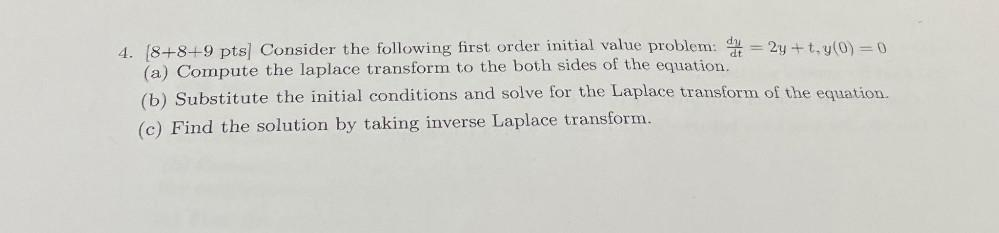Question:

# Consider the following first order initial value problem: dy/dt= = 2y +t, y(0) = 0 (a) Compute the laplace transform to the both sides of the equation. (b) Substitute the initial conditions and solveConsider the following first order initial value problem: dy/dt= = 2y +t, y(0) = 0 (a) Compute the laplace transform to the both sides of the equation. (b) Substitute the initial conditions and solve for the Laplace transform of the equation. (c) Find the solution by taking inverse Laplace transform.